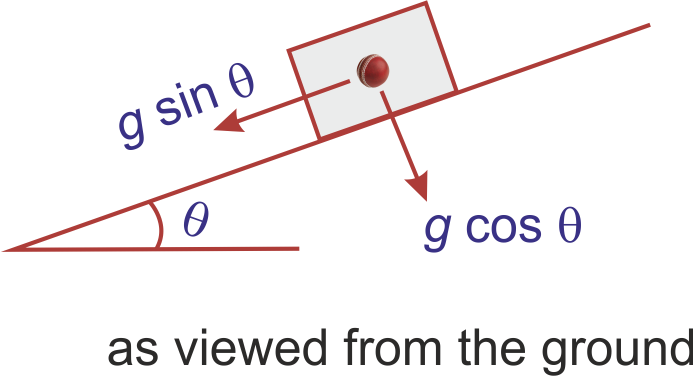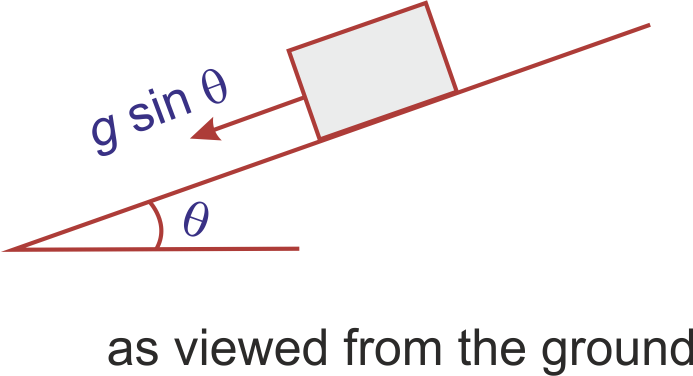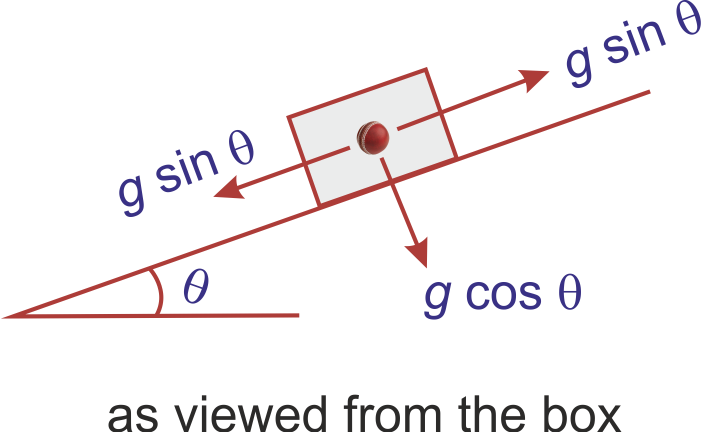## 1998 (8 marks)

A large heavy box is sliding without friction down a smooth plane of inclination θ. From a point P on the bottom of the box, a particle is projected inside the box. The initial speed of the particle with respect to the box is u and the direction of projection makes an angle α with the bottom as shown in the figure.
(a) Find the distance along the bottom of the box between the point of projection P and the point Q where the particle lands (Assume that the particle does not hit any other surface of the box. Neglect air resistance.)
(b) If the horizontal displacement of the particle as seen by an observer on the ground is zero, find the speed of the box with respect to the ground at the instant when the particle was projectedSolution: (a) Let's work in the frame for which the box is at rest, the acceleration of the ball being $$g cos \theta$$ normally downwards to the inclineThus this is the projectile motion in which g is replaced by $$g cos \theta$$. PQ is simply the range of projectile given by

$$PQ = \frac{u^{2}sin 2\alpha}{g cos \theta}$$.

(b) For an observer at ground, the acceleration of the projectile is g in vertically downward direction and no acceleration in horizontal direction. If the horizontal displacement is zero, it means horizonatal component of velocity is always zero. That simply indicates $$u cos (\alpha +\theta) = v cos\theta$$, where v is the velocity of the box down the incline. This gives

$$v = \frac{u cos (\alpha +\theta)}{cos\theta}$$.